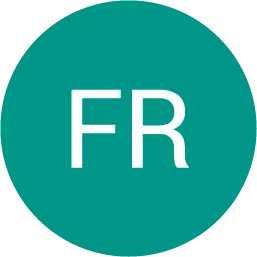, 22.06.2019 09:30 yasarhan2

# Here is a flower made up of yellow hexagons, red trapezoids, and green triangles. how many copies of this flower pattern could you build if you had 30 yellow hexagons, 50 red trapezoids, and 60 green triangles?### Another question on MathematicsMathematics, 21.06.2019 16:00
The equation x2 – 1x – 90 = 0 has solutions {a, b}. what is a + b?Mathematics, 21.06.2019 21:10
The vertices of a triangle are a(7.5), b(4,2), and c19. 2). what isMathematics, 21.06.2019 21:50
Which equation shows the quadratic formula used correctly to solve 5x2 + 3x -4 0 for x? cos -3+ v (3) 2-4() 2(5) 3+ |(3)² +4() 205) 3+ (3) 2-4() -3+ v (3)² +4()Mathematics, 21.06.2019 22:00
Jayne is studying urban planning and finds that her town is decreasing in population by 3% each year. the population of her town is changing by a constant rate.true or false?
Here is a flower made up of yellow hexagons, red trapezoids, and green triangles. how many copies of...
QuestionsQuestions on the website: 13977080